# scipy.spatial.distance.dice¶

scipy.spatial.distance.dice(u, v)[source]

Computes the Dice dissimilarity between two boolean 1-D arrays.

The Dice dissimilarity between u and v, is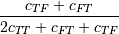where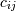is the number of occurrences of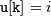and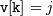for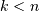.

Parameters: u : (N,) ndarray, bool Input 1-D array. v : (N,) ndarray, bool Input 1-D array. dice : double The Dice dissimilarity between 1-D arrays u and v.

#### Previous topic

scipy.spatial.distance.cosine

#### Next topic

scipy.spatial.distance.euclidean